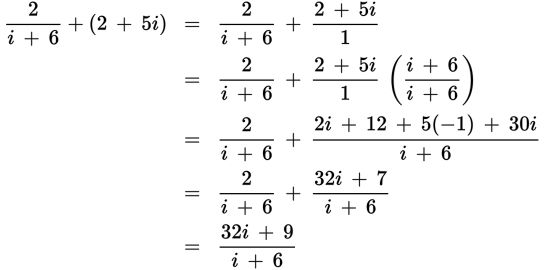# SAT Math Multiple Choice Question 269: Answer and Explanation

### Test Information

Question: 269

14. Which of the following expressions is equivalent to the complex number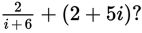(Note that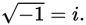)

• A.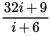• B.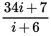• C.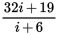• D.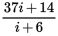Explanation:

A

Difficulty: Hard

Category: Additional Topics in Math / Imaginary Numbers

Strategic Advice: Fractions with complex numbers are no different from any other fraction. You must find a common denominator before adding them.

Getting to the Answer: Find a common denominator by multiplying the second term by i + 6. You're given that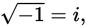but a more useful fact is that i2 = -1, so be sure to make this substitution as you go. Once you have found the common denominator, you can simply add like terms.